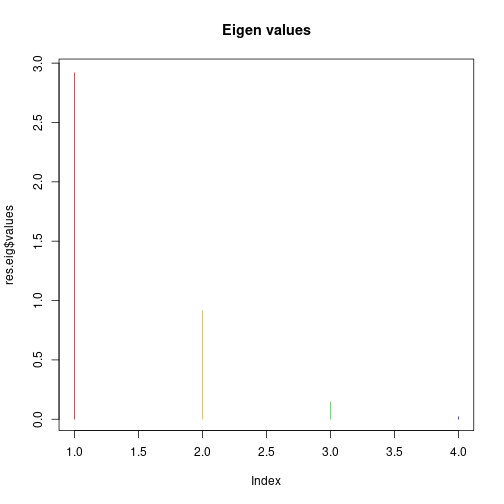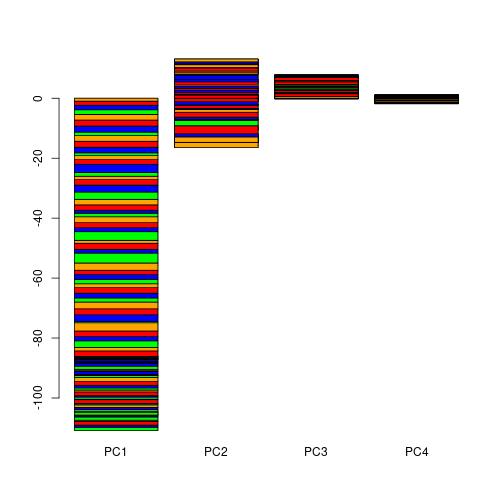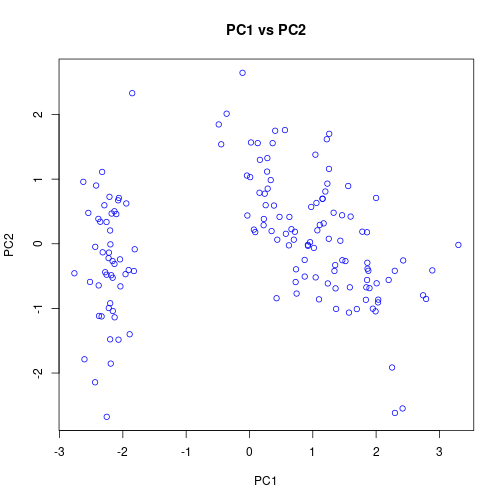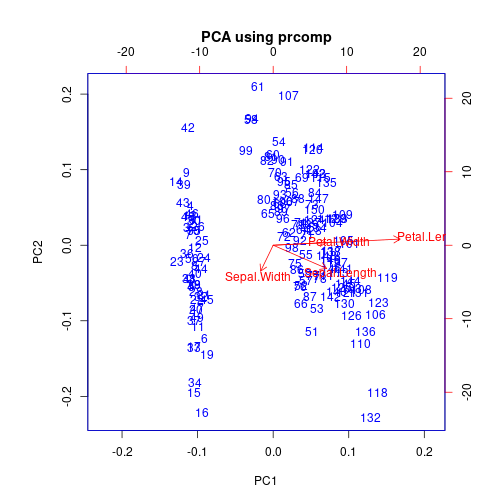# Principal component analysis (PCA) using R

0
3562

Principal component analysis (PCA) is a statistical analysis technique that uses an orthogonal transformation to convert a set of observations of possibly correlated variables into a set of values of linearly uncorrelated variables called principal components. Moreover, it has a wide variety of application in machine learning, it can be used to find structure in features and some pre-processing of the machine learning model.
Overall PCA is an ideal candidate to visualize the data along with the reduction of a number of data dimensions.

### Data preparation

```#Only Four columns (Sepal.Length Sepal.Width Petal.Length Petal.Width)
data = iris[,c(1,2,3,4)]
class(data)
```
```##  "data.frame"
```

### 1. Scale data

```data.scaled = scale(data, center = TRUE, scale = TRUE)
```
```##      Sepal.Length Sepal.Width Petal.Length Petal.Width
## [1,]   -0.8976739  1.01560199    -1.335752   -1.311052
## [2,]   -1.1392005 -0.13153881    -1.335752   -1.311052
## [3,]   -1.3807271  0.32731751    -1.392399   -1.311052
## [4,]   -1.5014904  0.09788935    -1.279104   -1.311052
## [5,]   -1.0184372  1.24503015    -1.335752   -1.311052
```

### 2. The correlation matrix

```res.cor <- cor(data.scaled)
res.cor
```
```##              Sepal.Length Sepal.Width Petal.Length Petal.Width
## Sepal.Length    1.0000000  -0.1175698    0.8717538   0.8179411
## Sepal.Width    -0.1175698   1.0000000   -0.4284401  -0.3661259
## Petal.Length    0.8717538  -0.4284401    1.0000000   0.9628654
## Petal.Width     0.8179411  -0.3661259    0.9628654   1.0000000
```

### 3. The eigenvectors of the correlation matrix

```res.eig <- eigen(res.cor)
res.eig
```
```## eigen() decomposition
## \$values
##  2.91849782 0.91403047 0.14675688 0.02071484
##
## \$vectors
##            [,1]        [,2]       [,3]       [,4]
## [1,]  0.5210659 -0.37741762  0.7195664  0.2612863
## [2,] -0.2693474 -0.92329566 -0.2443818 -0.1235096
## [3,]  0.5804131 -0.02449161 -0.1421264 -0.8014492
## [4,]  0.5648565 -0.06694199 -0.6342727  0.5235971
```
```plot(res.eig\$values, col=c("red","orange","green","blue"),type="h",main="Eigen values")
```As the first eigenvalue “2.91849782” is largest so it is our first principal component.

### 4. Let’s compute components by multiplying the transposed scaled matrix and transposed eigenvector matrix.

```# Transpose eigeinvectors
eigenvectors.t <- t(res.eig\$vectors)
data.scaled.t <- t(data.scaled)
# The new dataset
data.new <- eigenvectors.t %*% data.scaled.t
# Transpose new data ad rename columns
data.new <- t(data.new)
colnames(data.new) <- c("PC1", "PC2", "PC3", "PC4")
```
```##            PC1        PC2         PC3          PC4
## [1,] -2.257141 -0.4784238  0.12727962  0.024087508
## [2,] -2.074013  0.6718827  0.23382552  0.102662845
## [3,] -2.356335  0.3407664 -0.04405390  0.028282305
## [4,] -2.291707  0.5953999 -0.09098530 -0.065735340
## [5,] -2.381863 -0.6446757 -0.01568565 -0.035802870
## [6,] -2.068701 -1.4842053 -0.02687825  0.006586116
```
```barplot(data.new, col = c("red","orange","green","blue"))
``````plot(data.new, col = c("blue"), main="PC1 vs PC2")
```### PCA using prcomp function

```pca <- prcomp(iris[, -5])
summary(pca)
```
```## Importance of components:
##                           PC1     PC2    PC3     PC4
## Standard deviation     2.0563 0.49262 0.2797 0.15439
## Proportion of Variance 0.9246 0.05307 0.0171 0.00521
## Cumulative Proportion  0.9246 0.97769 0.9948 1.00000
```
```biplot(pca, col = c("blue","red"),main = "PCA using prcomp")
```https://www.rdocumentation.org/packages/stats/versions/3.5.3/topics/prcomp

Advertisement